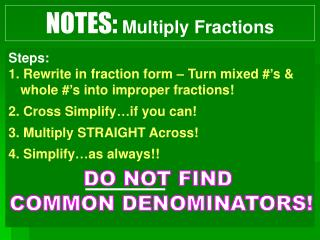DownloadDownload PresentationNOTES: Multiply Fractions

# NOTES: Multiply Fractions

Télécharger la présentation## NOTES: Multiply Fractions

- - - - - - - - - - - - - - - - - - - - - - - - - - - E N D - - - - - - - - - - - - - - - - - - - - - - - - - - -
##### Presentation Transcript

1. NOTES: Multiply Fractions • Steps: • 1. Rewrite in fraction form – Turn mixed #’s & whole #’s into improper fractions! • 2. Cross Simplify…if you can! • 3. Multiply STRAIGHT Across! • 4. Simplify…as always!! DO NOT FIND COMMON DENOMINATORS!

2. EXAMPLES: Multiply Fractions 1 1 2 2 3

3. EXAMPLES: Multiply Fractions 1 4 2 19 5 1 3 3 6 2 1

4. At the school dance, of the students wore the school colors. ofthem were in the 7th grade. What fraction of the students at the school dance wore the school colors and were in the 7th grade? A) B) C) D)

5. NOTES: Multiply Fractions with Models Steps: 1. Think of a fraction model for each factor. 2. Overlap those models, and that’s it!  3. The boxes that have both patterns represent the product. Example:

6. REVIEW: Multiply Fractions with Models What is one-half of one-third? We have : And we take half of it! MORE PRACTICE: http://www.learner.org/courses/learningmath/number/session9/part_a/try.html

7. Divide Fractions

8. NOTES: Divide Fractions with Models means how many ’s are in ? So, I have 4 groups of 2! Let’s try a fraction: • means how many ’s are in ? • So, I have 8 groups of ½!

9. NOTES: Dividing Fractions DO NOT Have to FIND COMMON DENOMINATORS! VOCAB: The product of a fraction and its reciprocal (or multiplicative inverse) is 1. …To make a reciprocal, flip the fraction upside down! Rewrite in fraction form – Turn mixed #’s & whole #’s into improper fractions! 2. Multiply by the reciprocal. **Keep It – Change It – Flip It Method 3. As always…SIMPLIFY!

10. NOTES: Dividing Fractions Multiplying Fractions, Ain’t no problem! Top times Top, and Bottom times Bottom. Dividing Fractions, Don’t be shy! Flip the second And multiply.

11. EXAMPLES: Dividing Fractions 1 2 1 3 1

12. EXAMPLES: Dividing Fractions 1 4 3 1 5 19 5 6 4

13. **If you want to find common denominators, you can. Your denominators will divide away, and you will divide the numerators straight across. Once you have a common denominator, divide straight across. 3 ÷ 2 =